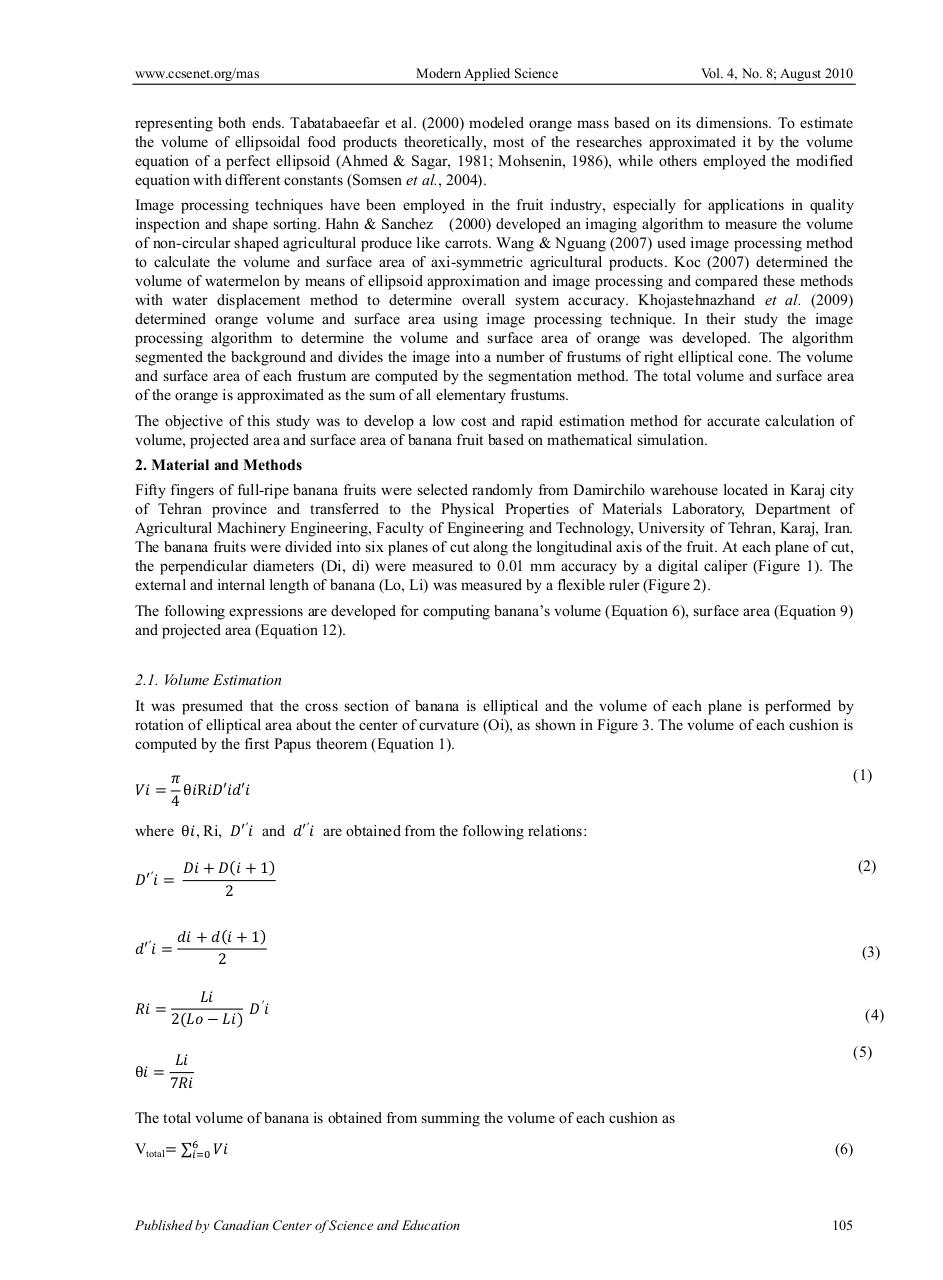# 5528.pdfPage 1 2 3 4 5 6 7 8 9 10

#### Text preview

www.ccsenet.org/mas

Modern Applied Science

Vol. 4, No. 8; August 2010

representing both ends. Tabatabaeefar et al. (2000) modeled orange mass based on its dimensions. To estimate
the volume of ellipsoidal food products theoretically, most of the researches approximated it by the volume
equation of a perfect ellipsoid (Ahmed &amp; Sagar, 1981; Mohsenin, 1986), while others employed the modified
equation with different constants (Somsen et al., 2004).
Image processing techniques have been employed in the fruit industry, especially for applications in quality
inspection and shape sorting. Hahn &amp; Sanchez (2000) developed an imaging algorithm to measure the volume
of non-circular shaped agricultural produce like carrots. Wang &amp; Nguang (2007) used image processing method
to calculate the volume and surface area of axi-symmetric agricultural products. Koc (2007) determined the
volume of watermelon by means of ellipsoid approximation and image processing and compared these methods
with water displacement method to determine overall system accuracy. Khojastehnazhand et al. (2009)
determined orange volume and surface area using image processing technique. In their study the image
processing algorithm to determine the volume and surface area of orange was developed. The algorithm
segmented the background and divides the image into a number of frustums of right elliptical cone. The volume
and surface area of each frustum are computed by the segmentation method. The total volume and surface area
of the orange is approximated as the sum of all elementary frustums.
The objective of this study was to develop a low cost and rapid estimation method for accurate calculation of
volume, projected area and surface area of banana fruit based on mathematical simulation.
2. Material and Methods
Fifty fingers of full-ripe banana fruits were selected randomly from Damirchilo warehouse located in Karaj city
of Tehran province and transferred to the Physical Properties of Materials Laboratory, Department of
Agricultural Machinery Engineering, Faculty of Engineering and Technology, University of Tehran, Karaj, Iran.
The banana fruits were divided into six planes of cut along the longitudinal axis of the fruit. At each plane of cut,
the perpendicular diameters (Di, di) were measured to 0.01 mm accuracy by a digital caliper (Figure 1). The
external and internal length of banana (Lo, Li) was measured by a flexible ruler (Figure 2).
The following expressions are developed for computing banana’s volume (Equation 6), surface area (Equation 9)
and projected area (Equation 12).
2.1. Volume Estimation
It was presumed that the cross section of banana is elliptical and the volume of each plane is performed by
rotation of elliptical area about the center of curvature (Oi), as shown in Figure 3. The volume of each cushion is
computed by the first Papus theorem (Equation 1).
4

(1)

θR

where θ , Ri,

and

are obtained from the following relations:
(2)

1

2
1

(3)

2

2

(4)
(5)

θ

7

The total volume of banana is obtained from summing the volume of each cushion as
Vtotal